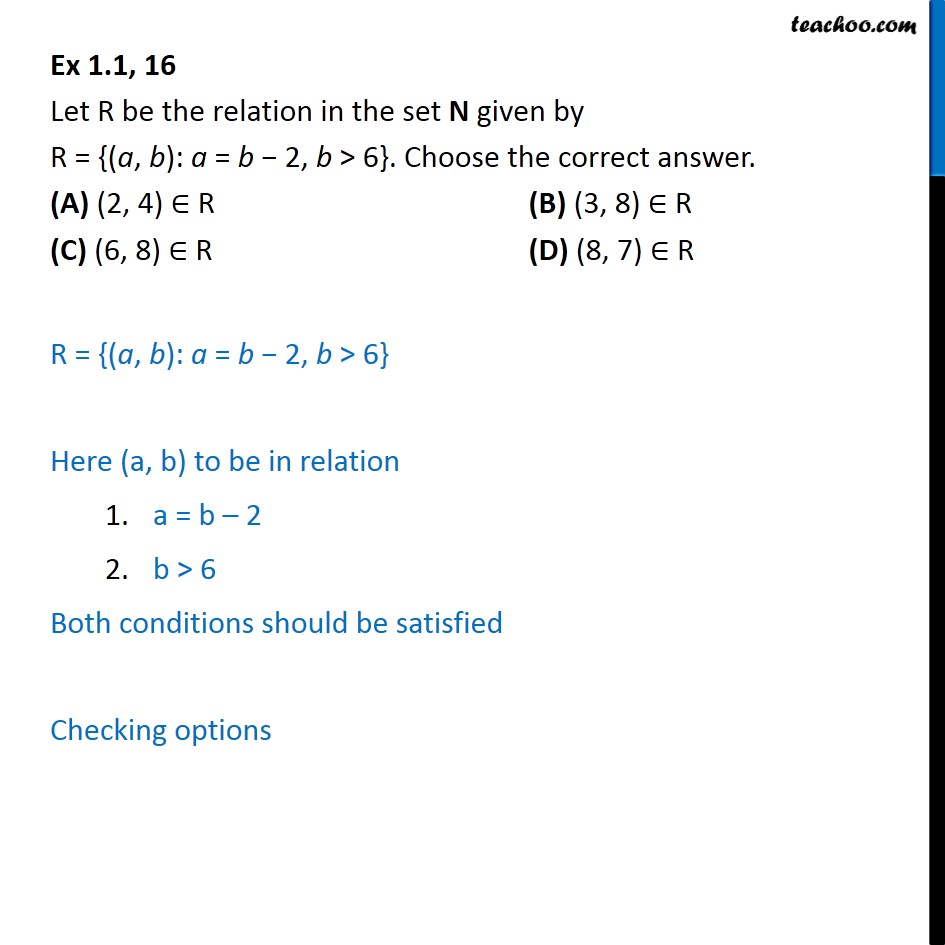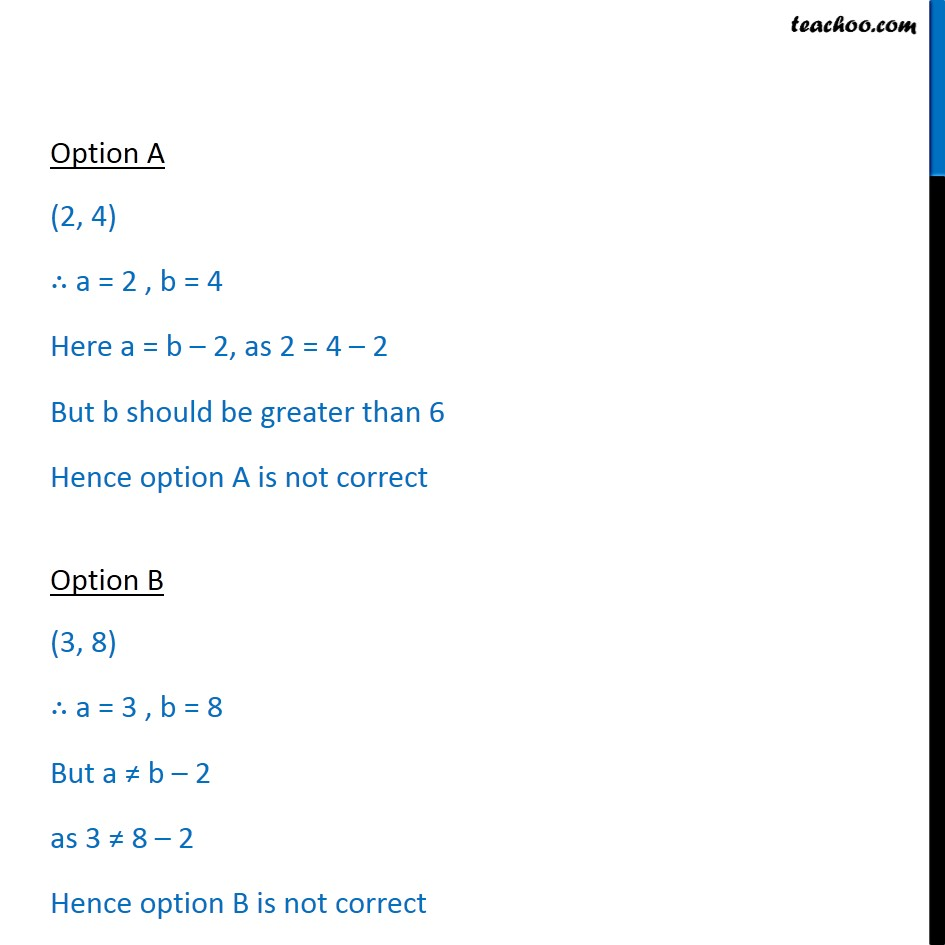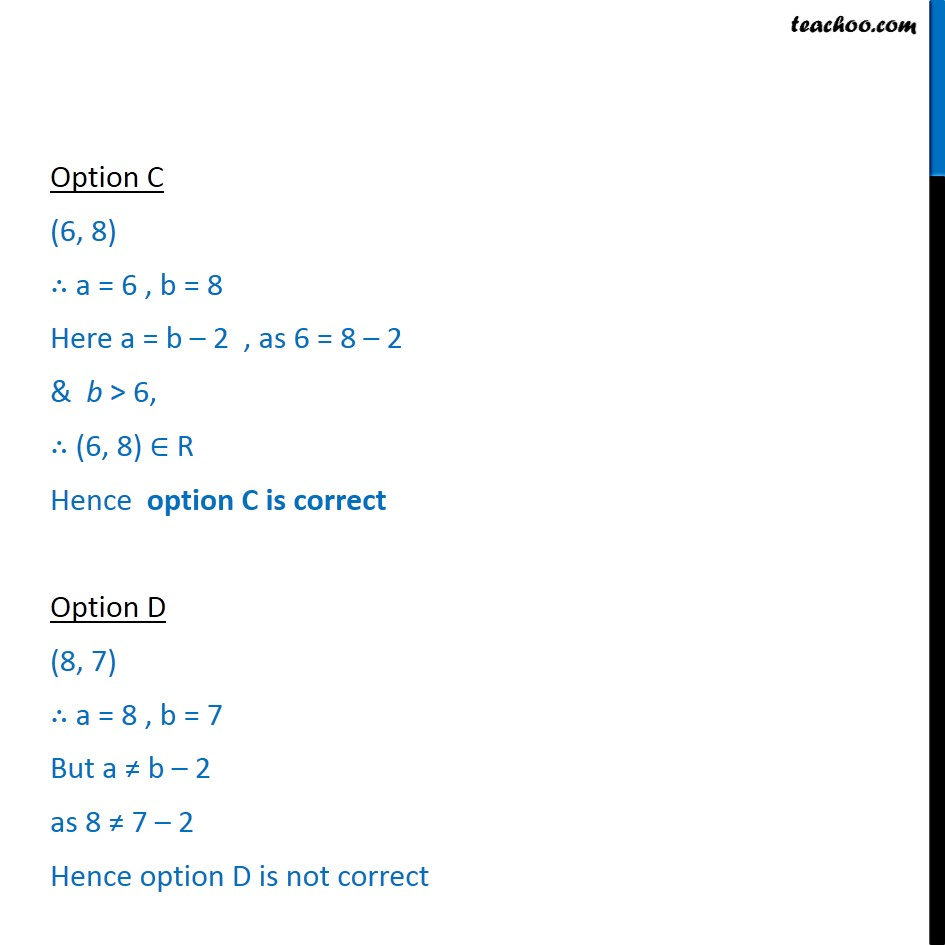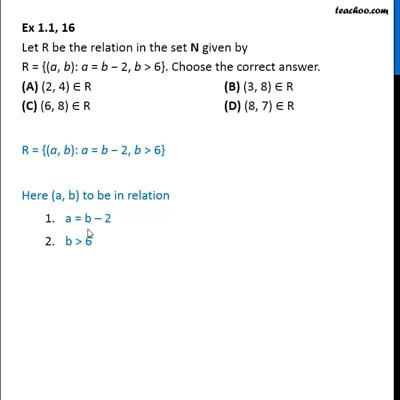Ex 1.1

Chapter 1 Class 12 Relation and Functions
Serial order wiseThis video is only available for Teachoo black users

Solve all your doubts with Teachoo Black (new monthly pack available now!)

### Transcript

Ex 1.1, 16 Let R be the relation in the set N given by R = {(a, b): a = b 2, b > 6}. Choose the correct answer. (A) (2, 4) R (B) (3, 8) R (C) (6, 8) R (D) (8, 7) R R = {(a, b): a = b 2, b > 6} Here (a, b) to be in relation a = b 2 b > 6 Both conditions should be satisfied Checking options Option A (2, 4) a = 2 , b = 4 Here a = b 2, as 2 = 4 2 But b should be greater than 6 Hence option A is not correct Option B (3, 8) a = 3 , b = 8 But a b 2 as 3 8 2 Hence option B is not correct Option C (6, 8) a = 6 , b = 8 Here a = b 2 , as 6 = 8 2 & b > 6, (6, 8) R Hence option C is correct Option D (8, 7) a = 8 , b = 7 But a b 2 as 8 7 2 Hence option D is not correct